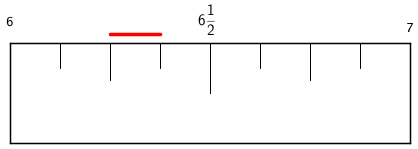### Home > MC1 > Chapter 3 > Lesson 3.4.5 > Problem3-147

3-147.

What is the distance on a ruler from $6\frac{1}{4}$to $6\frac{3}{8}$? If you need help, use your Giant Gazinch (from Lesson 2.1.3). Label your answer as inches.

This problem is very similar to problem 3-84, in which we refer to problem 2-20.
Use both of these problems as references if you are having difficulty.

Use the diagram below to help you find the distance between these two points on a ruler.